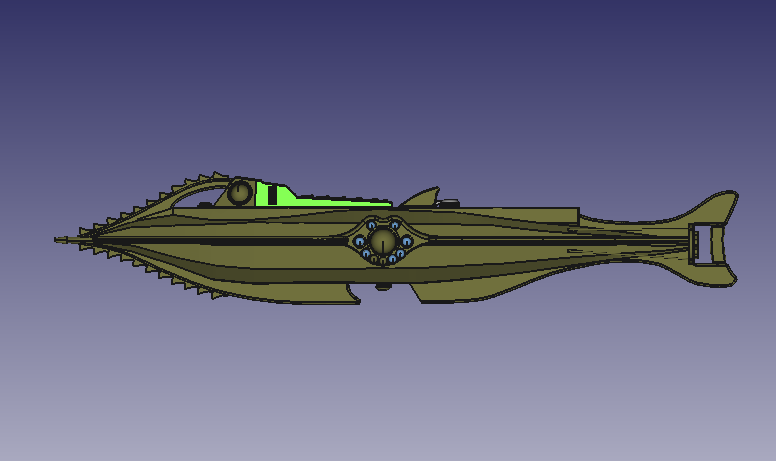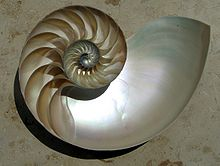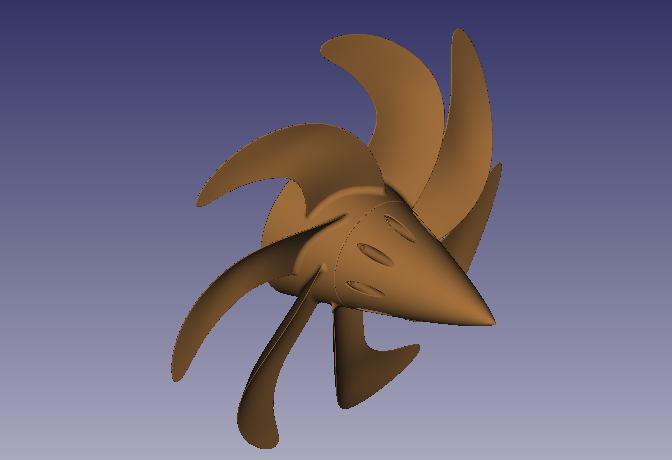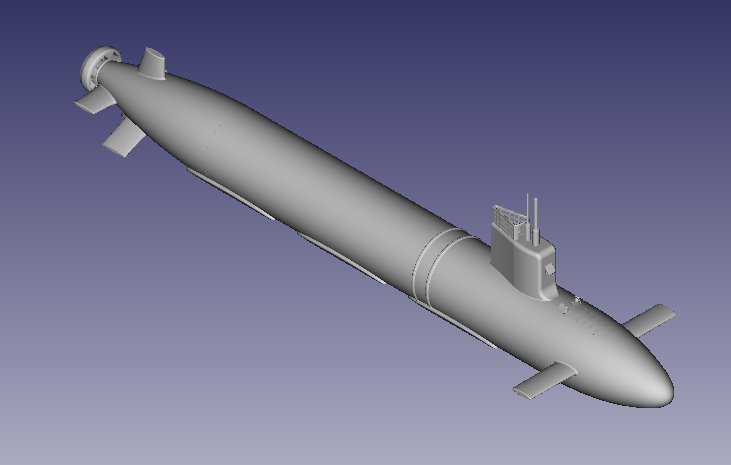2017-09-05

夏肇毅觀點:複製小抄大腦，讓金融科技搭起人工智慧塔

-若利率上漲，則債券下跌。
-若中東發生戰爭，則黃金石油大漲。

-若美國FED升息，那會有什麼影響?
-如果戰爭開打，那麼金價如何變動?

夏肇毅觀點:國防工業亟待人工智慧與雲端運算技術助力

AlphaGo Master同時並應用了目前人工智慧中最流行的CNN。噢，不是我們想的那個，而是「Convolution Neural Network」。一種當今最熱門，模仿人類大腦結構的人工智慧類神經網路，照片識別、語音秘書、自動駕駛都靠它。數十年來沒什麼進展的類神經網路，拜雲端運算所提供的大量運算能量之賜，這幾年突然又掀起了一股熱潮。

2017-09-04

Get Technical Analysis Indicators from BigObject In-Data Computing

Get Technical Analysis Indicators from BigObject In-Data Computing

BigObject In-Data Computing allows users to write their own program with LUA programming language. Similar to the store procedure concept of the SQL server, but a LUA script can do whatever users want to do together with the data stored in the BigObject. Like technical analysis applications of the financial market, BigObject can generate indicators like SMA,EMA,..etc. by walking thru data of the table with a LUA function ta().
The definition of SMA(simple moving average) is:
SMA(p,N)= ∑i=1,N pi / N

And the EMA(exponential moving average) is

EMA(p,N)i = α x pi + (1-α) x EMA(p,N)i-1
α=2/(N-1)

Convert these formulas into LUA we can get the attached sample code.

When we want to run this TA function, we simply use the APPLY command to call it up like:
APPLY ta('sma','stock','Close','ma', 120, 0,0,0,0)
APPLY ta('ema','stock','Close','ma', 120, 0,0,0,0)
APPLY ta('var','stock','Close','ma', 120, 0,0,0,0)
APPLY ta('sd','stock','Close','ma', 120, 0,0,0,0)

Source Code:

function ta(fun,v1,v2,v3,v4,v5,v6,v7,v8)
function sma(bt, col, rt_col, n)
t = bo.getTable(bt)
rt = t:getColumnValue(col);
for i = 2, #rt do
s=0.0
cnt=0
for j = 0, n-1 do
if (i-j>0) then
s = s + rt[i-j]
cnt=cnt+1.0
end
end
rt[i] = s/cnt
end
t:setColumnValue(rt_col, rt)
end

function ema(bt, col, rt_col, n)
t = bo.getTable(bt)
rt = t:getColumnValue(col);
alpha=2/(N+1)
for i = 2, #rt do
rt[i] = alpha*rt[i]+(1-alpha)*rt[i-1]
end
t:setColumnValue(rt_col, rt)
end

function var(bt, col, rt_col, n)
t = bo.getTable(bt)
rt = t:getColumnValue(col);
for i = 2, #rt do
s=0.0
cnt=0
for j = 0, n-1 do
if (i-j>0) then
s = s + rt[i+j]
cnt=cnt+1.0
end
end
av = s/cnt
s=0.0
cnt=0
for j = 0, n-1 do
if (i-j>0) then
s = s + math.pow((rt[i-j] - av),2)
cnt=cnt+1.0
end
end
rt[i] = s/cnt

end
t:setColumnValue(rt_col, rt)
end

function sd(bt, col, rt_col, n)
t = bo.getTable(bt)
rt = t:getColumnValue(col);
for i = 2, #rt do
s=0.0
cnt=0
for j = 0, n-1 do
if (i-j>0) then
s = s + rt[i-j]
cnt=cnt+1.0
end
end
av = s/cnt
s=0.0
cnt=0
for j = 0, n-1 do
if (i-j>0) then
s = s + math.pow((rt[i-j] - av),2)
cnt=cnt+1.0
end
end
rt[i] = math.pow(s/cnt,0.5)

end
t:setColumnValue(rt_col, rt)
end

if (fun=='sma') then
sma(v1,v2,v3,v4)
elseif (fun==’ema’) then
var(v1,v2,v3,v4)
elseif (fun=='var') then
var(v1,v2,v3,v4)
elseif (fun=='sd') then
sd(v1,v2,v3,v4)
end
end

夏肇毅觀點：當金融科技揮舞「人工智慧」利劍砍向傳統金融

• SWIFT正式選用Hyperledger，與3大金融機構聯手進行區塊鏈跨國交易驗證
• 3大變革Visa引領行動支付向前行
• 開放架構建立行動支付生態系
• 結合Fintech新創發展多元支付樣貌
• 預見需求優化消費者支付體驗

夏肇毅觀點：施董的體悟──創業最重要的是要氣長

f=(bp-q)/b

• f為現有資金應進行下次投注的比例
• b為投注可得的賠率（不含本金）
• p為獲勝率
• q為落敗率，即1-p

f=(20x0.1-0.9)/20=(2-0.9)/20=5.5%

夏肇毅觀點：卓董示警-台灣製造業5到10年將面臨淘汰危機

「日薄西山」製造業比想像的差:
●大量西進後的空洞化
●缺乏持續研發及升級投資，內外均陷困頓
●大學及研發法人追求空洞的SCI論文KPI

●連台大也淪陷的SCI(SSCI)論文症候群
-每年發表超過25,000篇SCI論文，成為美國(先進工業國家)的「研究代工中心」，有「產量」而少「產值」
-政府科專計劃也深受SCI論文主導，有「投入」而少「產出」。缺乏產業效益，資源正逐漸枯竭(稅基流失)，政府即將難以為繼
●這些SCI論文，和台灣的國家競爭力有甚麼關係？

●碩果僅存的2.0製造業若不能升級，5年後將全遭淘汰(水五金、手工具、粉末冶金、鑄造品、五金製品...)
●3.0的製造業若不能升級，10年後就岌岌可危
●「除非你先做好一個中尉，否則你做不到將軍」-克林鮑威爾，前美國國務卿

夏肇毅觀點：潛艦國造啟動自主深海探索元年(from GrabCAD, 小說電影中鸚鵡螺號模型Nemo by john    圖片製作:夏肇毅)(from wiki, 鸚鵡螺與其殼室by Manuae,Chris73)(from GrabCAD Submarine Propeller模型 by M.B.I. 圖片製作:夏肇毅)(from GrabCAD 美國Minnesota潛艇模型 by Asm Imam 圖片製作:夏肇毅)